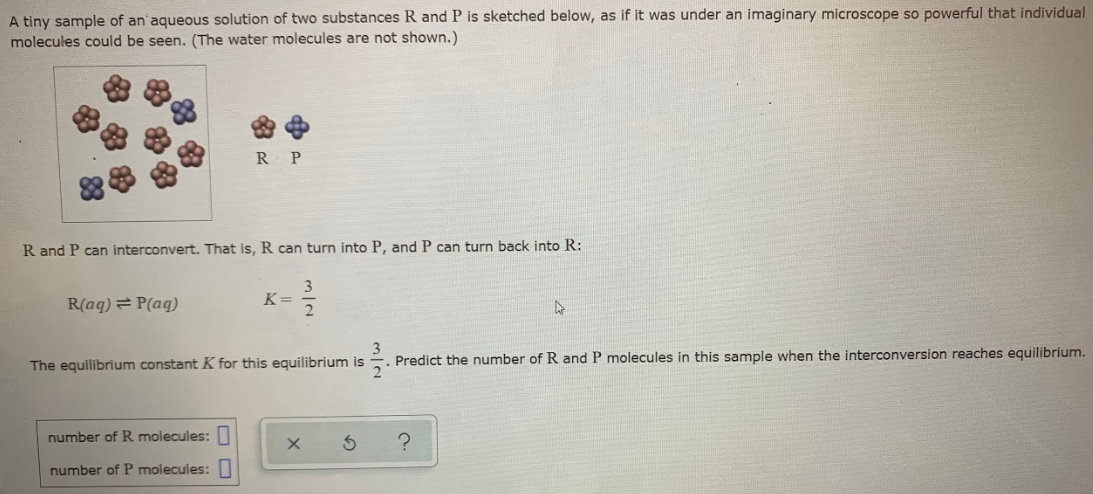# A tiny sample of an aqueous solution of two substances R and P is sketched below, as if it was under an imaginary microscope so powerful that individual molecules could be seen. (The water molecules are not shown.) R and P can interconvert. That is, R can turn into P, and P can turn back into R: R(aq) ⇌ P(aq) K = 3/2. The equilibrium constant K for this equilibrium is 3/2. Predict the number of R and P molecules in this sample when the interconversion reaches equilibrium